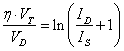# Electronics I Laboratory 3

Today we will complete the first-cut audio amplifier and measure the v-i curve for a diode.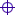Two Op-Amp Amplifier with Complementary Transistor DriverSchematic of Two Op-Amp Differential Driver Circuit with Complementary OutputDiode v-i Curve ExperimentSchematic of Diode v-i Curve Test CircuitInstructions for your Report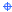Report Instructions for Two Op-Amp Amplifier with Complementary Transistor DriverReport Instructions for Diode v-i Curve Experiment

## Two Op-Amp Amplifier with Complementary Transistor DriverBeginning with the Complementary Transistor Driver circuit form Laboratory 2, add the two op-amp driver circuit to make up a complete circuit as shown below as Figure 1.  Replace the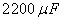capacitor and speaker at the output with a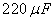capacitor and a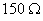load for today's laboratory.  If you used 2N3904/2N3906 instead of 2N4401/2N4403, leave your transistors as placed in the circuit for this laboratory.  You may be given an LM358, a dual op-amp, instead of an LM324, a quad op-amp.  If you get an LM324, ask the Instructor how to hook up the unused op-amps.Measurements of voltage that assure that the circuit is working are given below in the report instructions.  Make these measurements after your circuit is working and record them for your report.Set up your signal generator for a sine wave outputAmplitude 1 millivolt peakFrequency 1,000 HzApply the amplifier and signal generator output to the oscilloscope.  The amplifier output is the voltage across theload.  Adjust the signal generator output as necessary to provide as large a s possible signal at the output without visible distortion.  Measure the voltage gain of the circuit.Save the plots for your lab reportRepeat for a 1 millivolt peak triangular wave at 1,000 Hz.Repeat for a 1 millivolt peak square wave at 1,000 Hz.Change the frequency of the signal generator to find the high and low frequency limits over which the output amplitude remains approximately constant.  Write down the frequencies for which the amplitude of the output is 0.7 times the amplitude at 1000 Hz.  These are the 3 dB points in the frequency response.

## Schematic of Two Op-Amp Differential Driver Circuit with Complementary Output

Note that this circuit is a circuit involving two op-amps, added to the circuit built for Laboratory 1.   Leave the circuit for Laboratory 3 on the wireless prototype board because you will be using it when we discuss biasing of three-terminal devices and for other experiments.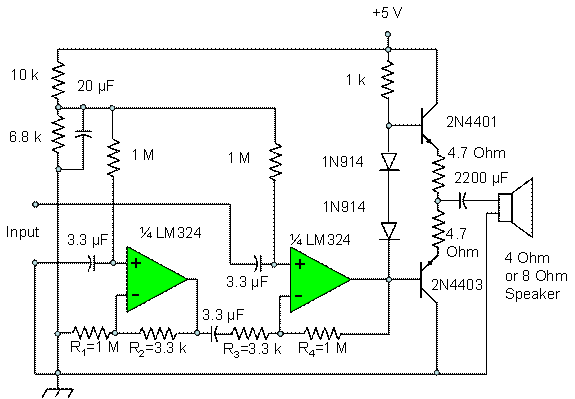Figure 1.  Completed First-Cut Audio Amplifier

## Diode v-i Curve ExperimentConstruct the circuit consisting of a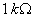resistor in series with a diode as shown in Figure 2.  While the resistor is not connected to the power supply, measure its resistance with your multimeter to the highest accuracy possible with the instrument.  Record this resistance for use in finding currents, and for your report.Adjust your power supply for voltages between 0 V and 6 V and measure the voltage and current through the diode.  Measure the current by connecting your multimeter across the resistor and recording the voltage drop, then divide by the resistance that you measured.  Note that the ground of the multimeter must be floating, not connected to bench ground, for this measurement.  Use, as a minimum, the voltages shown in Table 1.

Table 1.  Voltages to be Applied to Diode v-i Test Circuit.  Measurements to be Taken are Diode Voltage and Current.

 Power Supply voltage Diode Voltage Diode Current 0.1 Volts 0.2 Volts 0.3 Volts 0.4 Volts 0.5 Volts 0.6 Volts 0.7 Volts 0.8 Volts 0.9 Volts 1.0 Volts 1.5 Volts 2.0 Volts 2.5 Volts 3.0 Volts 3.5 Volts 4.0 Volts 4.5 Volts 5.0 Volts 5.5 Volts 6.0 Volts

## Schematic of Diode v-i Curve Test Circuit

This is a simple circuit for measuring the voltage and current through a diode, and varying it over input currents up to about 5 milliamperes.  Make sure that your diode is inserted with the cathode to ground.  Check by applying about 2 Volts to the circuit and checking the output voltage; if the output voltage is about 2 volts, reverse the diode.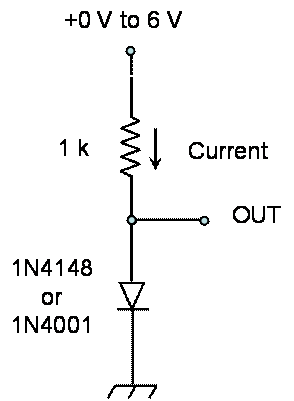Figure 2 Circuit for Measuring Diode v-i Curve

Use the instructions given on this link.  The lab report is due one week from today.  Submit your report as a file by e-mail to no_spam_jkbeard@jameskbeard.com.

### Report Instructions for Two Op-Amp Amplifier with Complementary Transistor DriverMeasure the DC voltage at the voltage divider at the top left of the circuit.  This voltage divider is a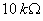and a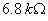resistor in series, with a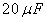capacitor bypassing theresistor.  This voltage should be about 2.02 Volts.  This is your circuit reference voltage.Measure the DC voltage at the inverting inputs to both op-amps.  Both should be the same as that of the voltage divider.  In your report, explain why this is so.Measure the voltage at the output of the left op-amp in the circuit.  It should be slightly more than the circuit reference voltage.  Explain why in your report.The first op-amp we define here is the leftmost in the schematic.  This op-amp is a non-inverting amplifier for the input at the lower of the two input terminals.  Find the gain of this non-inverting amplifier as a function of the two resistors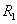andon the first op-amp, up to the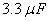coupling capacitor.The second op-amp we define here is the rightmost in the schematic.  To the signal from the first op-amp, this is an inverting amplifier.  Find the gain of this inverting amplifier as a function of the two resistors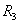and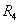in this op-amp circuit.The first op-amp circuit is a non-inverting amplifier for the upper of the two input terminals.  Find th gain of this non-inverting amplifier as a function of the two resistorsandin this op-amp circuit.Show that ifand, then the output of the second op-amp is a gain factor times the difference between the voltages at the input terminals.  Find this gain factor in terms ofand.  Give the relationship between the values of the resistors,,andthat is required for the two op-amp circuits together to be a differential amplifier.Note that we are using 5% tolerance for the resistors,,and, so we cannot expect for the differential gain to be exact.  The difference between the gains is called the common mode gain.  Measure the common mode gain by connecting the two input terminals together and applying a 1000 Hz sine wave voltage to them with the signal generator.  Apply up to 2.5 volts peak to get a measurable signal on the output, across theload.  Note that you measured the gain of the circuit as part of the experiment; this is the differential gain.  Compare the common mode gain with the differential gain.  Give the difference as a voltage ratio and as a figure in dB.

### Report Instructions for Diode v-i Curve ExperimentPlot the diode v-i curve from the data you took in the experiment.Compare the v-i curve with a theoretical curve using the equation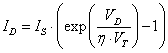whereWill changing the values of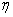and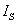give you better agreement?  What are these values?  Note that more data at the point where the current begins to flow can be helpful here.  Also, the v-i curve equation can be manipulated to give a form that may be better in understanding the roles ofandin the v-i curve.